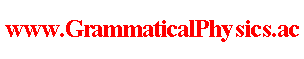since 2006 Help　Sitemap < Forum > < Problems > < Quantum History Theory > < Line Integral in Function Space > ξ(□, x) represents a function which is a point in the function space for each x. So, ξ is a parametric representation of a line in the function space, and x is a parameter of it. By thinking of t as an index of a coordinate in the function space, η(x) = √{α∫dt [∂ξ(t,x)/∂x]2} is understood as ds/dx where ds is the length of a line element. η(x) is chosen to be 1 in the definition of the probability formula. [dar(f; a, a + 1/α)]pre Φ[χ] = ∫dx η(x) f(x)* Φ[ξ(□,x)] = ∫dx f(x)* Φ[ξ(□,x)] --- This article is a rewrite of the article 'Line Integral in Function Space' in the following page. Later Edition@Theory of Quantum History Entangled in Time-like Direction@Products of Grammatical Physics@Grammatical Physics@Forum@Vintage(2008-2014) Author Yuichi Uda, Write start at 2015/05/21/20:06JST, Last edit at 2015/05/23/18:01JST